# Iterators in C++

## Iterators in C++ with Examples:

In this article, I am going to discuss Iterators in C++ with Examples. Please read our previous article where we discussed Containers in C++ with Examples. Iterators are a very important component of C++ STL. So, let us see about the iterators.

##### Iterators in C++

Iterators are the objects that enable us to traverse containers in some order for either reading or writing. Iterators are defined as templates in C++ and they must comply with a very specific set of rules in order to qualify as one of the many types of iterators. We will look at all the different types of iterators and what are the rules for them to qualify as that type of iterator.

One question that may come to your mind is are pointers and iterators the same thing. The short answer is no. let us see why. There are some things that are common to them. Pointer is variables and these can be indirected to refer to some memory location. A pointer however meets the requirements for many types of iterators. We can say that pointers are one particular type of iterator. The same happens with the integers. So, when acting as indices to arrays. These integers are iterators for that particular application but they are essentially discrete numeric values. So, integers also qualify as iterators of some type. In short, we can say that an iterator is a higher-level abstraction of elements that can be used to traverse containers whereas pointers and integers are a special type of iterator, not suitable for every container. Now let us look at different types of iterators.

##### Types of Iterators in C++:
1. Input Iterator
2. Output Iterator
3. Forward Iterator
4. Bidirectional Iterator
5. Random-access Iterator

There are 5 types of iterators in C++ and the order is important that we have listed these iterators. Input and output iterators are the ones with the smallest or least capabilities and these have the least requirements in order to implement them. We can clearly understand the working of these iterators by their name. We will look at them in detail. All the containers require their iterators to comply with the capabilities of some of these types. Some are rather relaxed; some are more rigorous. So, the first type is the input iterator.

##### Input Iterator in C++:

As the name says, these are input iterators that are only able to read. So, they can only access, but can-do assignments. If you have done some programming with iterators, you noticed that you can dereference them. So, either you write *it to dereference them or if the ‘it’ iterator is pointing to an object of some class then you can access the member values using the arrow operator i.e. it->val. These are two different ways of dereferencing an iterator or accessing the value through an iterator.

So ‘*it’ means we can only access it. If we write ‘*it = new_value’, this will not be allowed on the input iterator. It only moves in the forward direction and can be incremented. So you can do ‘it++’ or ‘++it‘. So, these can be accessed sequentially only. You cannot advance by more amount. ‘—it’ or ‘it—‘ are not allowed. It cannot go back; it can move only in the forward direction.

Only one pass is possible. If you require some algorithm when you need multiple passes then this would not be suitable for that case. And these are iterators along with output iterator which has the least requirement support needed for STL. So least capability is required to implement them. And these are suitable for input streams such as keyboard buffers or read-only files and very similar to this but kind of complementary to this is Output Iterator.

##### Output Iterator in C++:

This iterator is just able to write and not read. So here if you access it, let us say we have an integer value container ‘it’, ‘it = 10’. So, we can assign it to an integer. When we dereference it, it will be dereferenced as the L value. Whereas in the case of the input iterator, it was the R-value. So that’s why the input operator was not allowed to assign a new value.

It can move in forward direction only. So you can do ‘++’ but not ‘—‘. Here also only one pass is possible and the least requirement is similar to the input iterator. This is suitable for output streams such as screen text or write-only files. The next iterator is the forward iterator.

##### Forward Iterator in C++:

It has the requirement for both the input and the output iterator. But both input and output were moving in forward direction only. And it meets the requirement of both of these and this also moves in forward and it’s able to read and write both. So, you can dereference it. And either you can read it or write it both are allowed. It supports multiple passes of containers. But again it does not mean that you can do ‘—‘. This is not allowed.

It is suitable for traversing a singly linked list. Since in a singly linked list, you only need to move forward not backward. So, you do not need this capability to move backward. So, you can straight relate that if we have input, output, and forward iterator, so you need to inherit from input and output iterator. You combine the capabilities of the input and output iterator and you can form a forward iterator. The next iterator is a Bidirectional iterator.

##### Bidirectional Iterator in C++:

It combines all the capabilities of forward iterator. So, this naturally means all the capabilities of input and output are also combined. It combines the capabilities of forward and additionally allows backward traversal. So here you can perform ‘++’ as well as ‘—‘. It is suitable for a doubly linked list where you may need to go forward as well as backward. You can notice that iterators are becoming more and more powerful now. The last iterator is the Random-Access iterator.

##### Random-Access Iterator:

It is the most versatile iterator. It combines the capabilities of all the previous iterators which is a bidirectional iterator and then it additionally provides random access by means of indexing. So random access was not present in any of those. These are suitable for vectors and arrays.

Now we can summarize all the iterators. Let us create a diagram,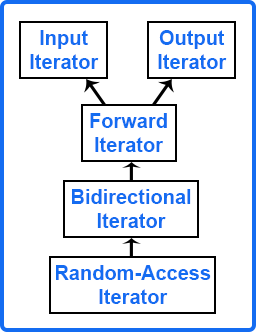##### Iterator Functions in C++:

Let us understand the different Iterator Functions in C++ with Examples. The first Iterator function that we are going to discuss is the begin() function.

##### Begin() Iterator Function in C++:

It returns the iterator to the beginning of the container. So, if we have vector v as follows: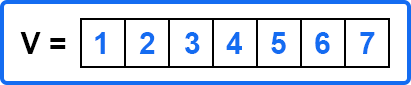And if we call v.begin(). This will return the iterator to the first element of the container. Let us say,
it = v.begin();
We can dereference it and also print it. So, if we print this value,
cin >> *it;
This will print 1. So, the begin iterator function in C++ points to the first element or the beginning of the container. Similarly, we have an end function.

##### End() Iterator Function in C++:

Unlike the begin iterator function, it does not point to the end of the container but instead, it returns an iterator to the element following the last element. So, 7 is the last element, and following this, assume there is an imaginary element that is actually not there in the container.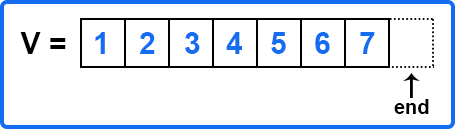So, the end iterator function in C++ will return an iterator pointing to this. The way to call this is also similar to the begin function.
it = v.end();
So, what if the container is empty? The container does not have any elements. You can think of it as a straight line. In this situation, begin will be the same as the end. So, if begin is the same as the end then the container will be empty. This element act as a placeholder; attempting to access it results in undefined behavior. We will see an example of this. What happens if we try to access or try to dereference it?

Dereferencing the begin() function is very simple. It is pointing to the first element so it should always return the first element. But in this case, it can return a different value. It is undefined. Depending on what is stored here at this location.

These functions are for forwarding iterators. Some containers also support reverse iterators. Not all the containers support it. For example, if we have a forward list that is a singly linked list and it is not expected to support a reverse iterator that is traversing from end to begin. Now let’s see those two other functions which are very similar to the begin and end functions.

##### Rbegin() Iterator Function in C++:

The Begin Iterator function returns the first element iterator to the first element. Similarly, the rbegin iterator function means we are dealing with reverse iterators, and reverse iterators traverse in the reverse direction.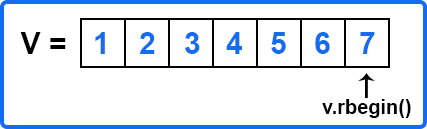It’s very similar to forward iterators where it will return an iterator pointing to the last element i.e. 7. So 7 is rbegin first element in the reverse direction which is the last element of the container. And if we dereference it then it will print 7.

##### Rend() Iterator Function in C++:

The Rend() iterator Function in C++ is very similar to rbegin you might have guessed that rend returns a reverse iterator pointing to the theoretical element right before the first element in the array container. The container address starts from 1st element.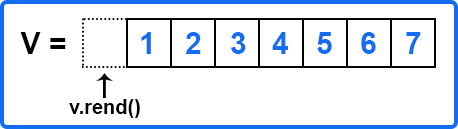If we assume there is a theoretical element before 1 then rend will point before the first element and again, we should not try to dereference this.

So now let us write the program where we will see examples of all the four functions that we have studied. We will select vector as an example where all four functions will be supported. Since we can traverse in the forward direction as well as the reverse direction.

##### Example to Understand Iterator Functions in C++:
```#include <iostream>
#include <vector>
using namespace std;

int main()
{
vector<int> v = {1, 2, 3, 4, 5, 6, 7};

cout << "v.begin() => " << *v.begin() << endl;
cout << "v.end() => " << *v.end() << endl;

cout << "v.rbegin() => " << *v.rbegin() << endl;
cout << "v.rend() => " << *v.rend() << endl;

return 0;
}
```
###### Output: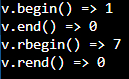0 is printed for after 7 and before 1 in the v vector. Let us add 30 after 7 in the array and check what will be printed.

```int main()
{
vector<int> v = {1, 2, 3, 4, 5, 6, 7};

int *i = &v;
i++;
*i = 30;

cout << "v.begin() => " << *v.begin() << endl;
cout << "v.end() => " << *v.end() << endl;

cout << "v.rbegin() => " << *v.rbegin() << endl;
cout << "v.rend() => " << *v.rend() << endl;

return 0;
}
```
###### Output: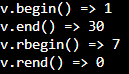Now v.end() has printed 30 but before it printed 0. The point that we are trying to cover is that you should not try to dereference it. It’s undefined. These are only used in certain algorithms. For example, let’s say you have inserted some keys in an unordered set and you want to check whether it’s present or not. Then you will use the find function and pass the key that you want to check inside the find function and then double equal sign then write the “SET_NAME.end()”. Suppose the set name is x, so the statement will be,

if (x.find(‘r’) == x.end()){
…
}

This means it has traversed the complete set and it went passed that where the end is pointing. These are useful for checks. So, you should not try to dereference it.